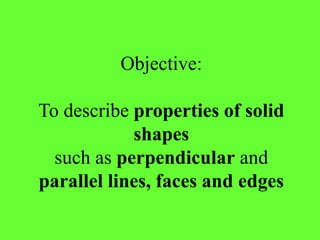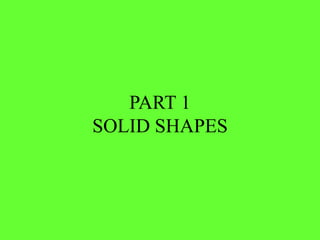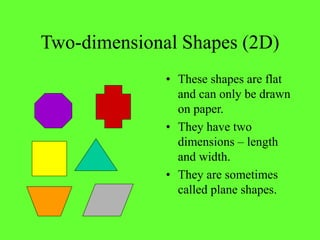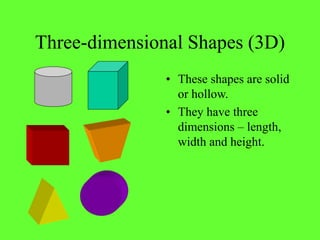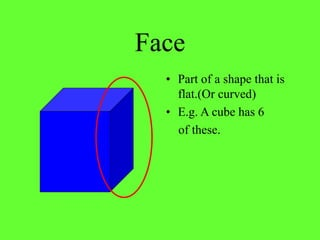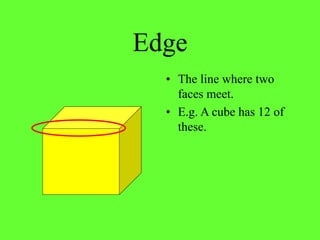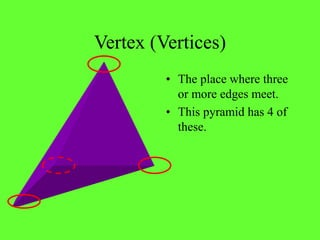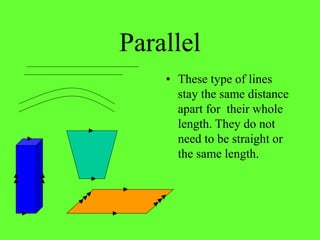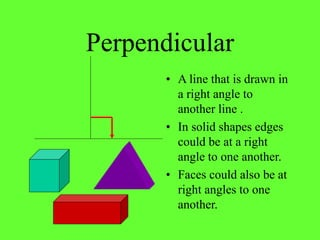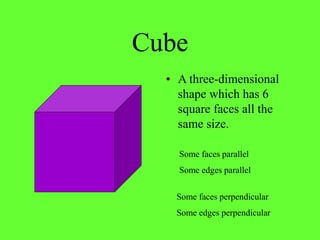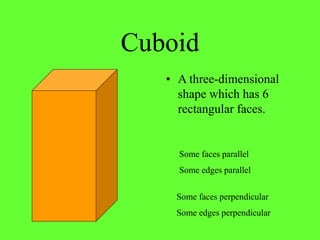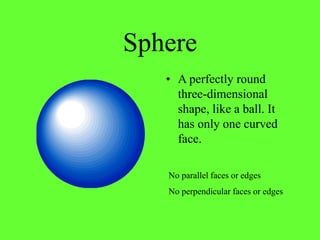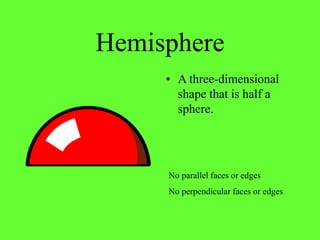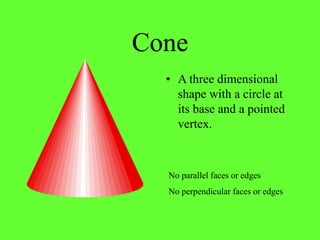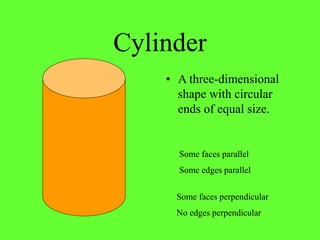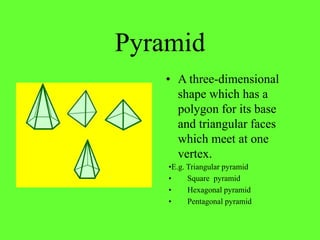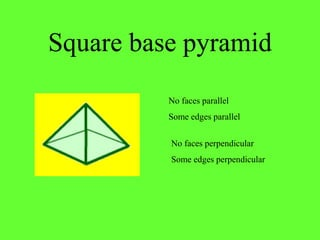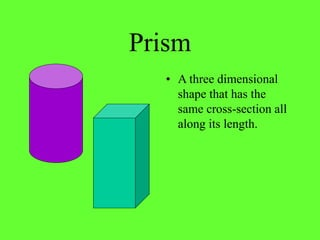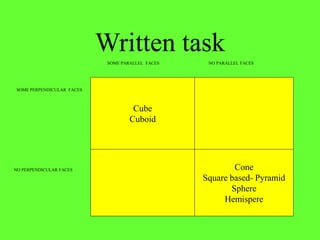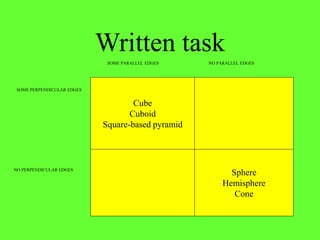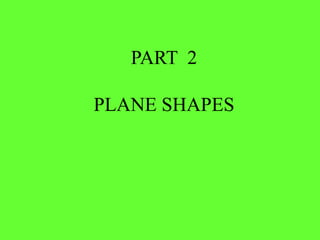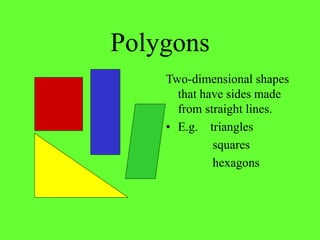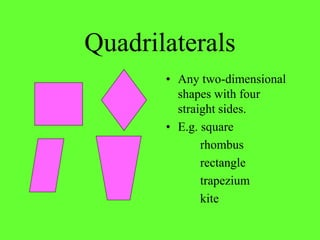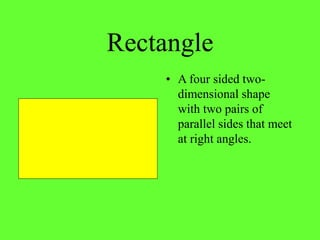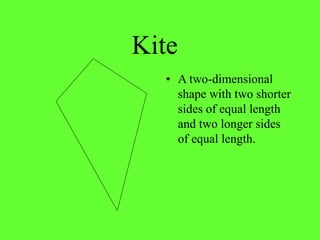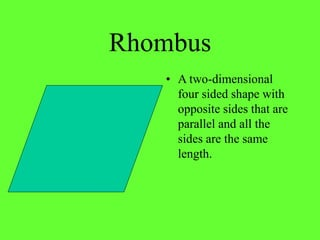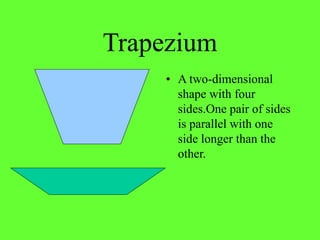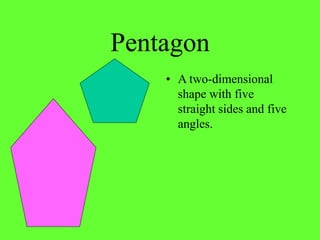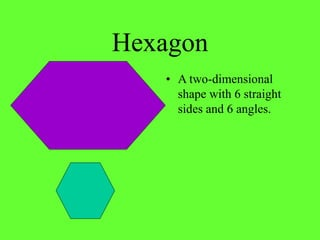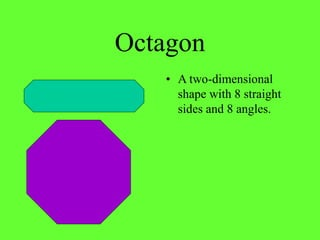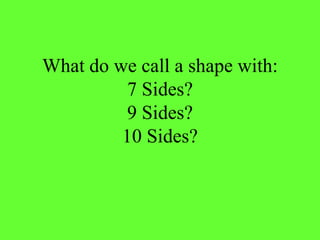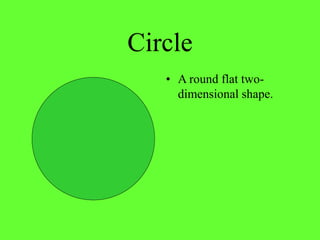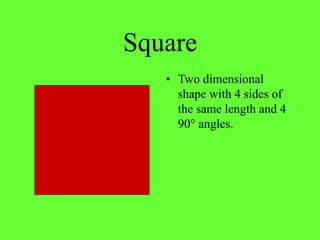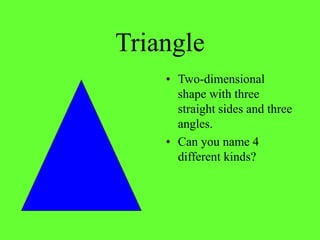1 de 34

### Properties of 2_d_3d_shapes

1. Objective: To describe properties of solid shapes such as perpendicular and parallel lines, faces and edges
2. PART 1 SOLID SHAPES
3. Two-dimensional Shapes (2D) • These shapes are flat and can only be drawn on paper. • They have two dimensions – length and width. • They are sometimes called plane shapes.
4. Three-dimensional Shapes (3D) • These shapes are solid or hollow. • They have three dimensions – length, width and height.
5. Face • Part of a shape that is flat.(Or curved) • E.g. A cube has 6 of these.
6. Edge • The line where two faces meet. • E.g. A cube has 12 of these.
7. Vertex (Vertices) • The place where three or more edges meet. • This pyramid has 4 of these.
8. Parallel • These type of lines stay the same distance apart for their whole length. They do not need to be straight or the same length.
9. Perpendicular • A line that is drawn in a right angle to another line . • In solid shapes edges could be at a right angle to one another. • Faces could also be at right angles to one another.
10. Cube • A three-dimensional shape which has 6 square faces all the same size. Some faces parallel Some edges parallel Some faces perpendicular Some edges perpendicular
11. Cuboid • A three-dimensional shape which has 6 rectangular faces. Some faces parallel Some edges parallel Some faces perpendicular Some edges perpendicular
12. Sphere • A perfectly round three-dimensional shape, like a ball. It has only one curved face. No perpendicular faces or edges No parallel faces or edges
13. Hemisphere • A three-dimensional shape that is half a sphere. No parallel faces or edges No perpendicular faces or edges
14. Cone • A three dimensional shape with a circle at its base and a pointed vertex. No perpendicular faces or edges No parallel faces or edges
15. Cylinder • A three-dimensional shape with circular ends of equal size. Some faces parallel Some edges parallel Some faces perpendicular No edges perpendicular
16. Pyramid • A three-dimensional shape which has a polygon for its base and triangular faces which meet at one vertex. •E.g. Triangular pyramid • Square pyramid • Hexagonal pyramid • Pentagonal pyramid
17. Square base pyramid No faces perpendicular Some edges perpendicular No faces parallel Some edges parallel
18. Prism • A three dimensional shape that has the same cross-section all along its length.
19. Written task Cube Cuboid Cone Square based- Pyramid Sphere Hemispere SOME PERPENDICULAR FACES NO PERPENDICULAR FACES SOME PARALLEL FACES NO PARALLEL FACES
20. Written task Cube Cuboid Square-based pyramid Sphere Hemisphere Cone SOME PERPENDICULAR EDGES NO PERPENDICULAR EDGES SOME PARALLEL EDGES NO PARALLEL EDGES
21. PART 2 PLANE SHAPES
22. Polygons Two-dimensional shapes that have sides made from straight lines. • E.g. triangles squares hexagons
23. Quadrilaterals • Any two-dimensional shapes with four straight sides. • E.g. square rhombus rectangle trapezium kite
24. Rectangle • A four sided two- dimensional shape with two pairs of parallel sides that meet at right angles.
25. Kite • A two-dimensional shape with two shorter sides of equal length and two longer sides of equal length.
26. Rhombus • A two-dimensional four sided shape with opposite sides that are parallel and all the sides are the same length.
27. Trapezium • A two-dimensional shape with four sides.One pair of sides is parallel with one side longer than the other.
28. Pentagon • A two-dimensional shape with five straight sides and five angles.
29. Hexagon • A two-dimensional shape with 6 straight sides and 6 angles.
30. Octagon • A two-dimensional shape with 8 straight sides and 8 angles.
31. What do we call a shape with: 7 Sides? 9 Sides? 10 Sides?
32. Circle • A round flat two- dimensional shape.
33. Square • Two dimensional shape with 4 sides of the same length and 4 90° angles.
34. Triangle • Two-dimensional shape with three straight sides and three angles. • Can you name 4 different kinds?